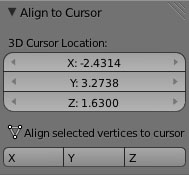# XYZ location of Vertices

This is something I come across often maybe someone can help me resolve. I am working on a model with vertices at various Z locations (ie 1.1, 1.3, 1.9… and so on). I want to give them all the same Z location (ie 1.2), but if I select each one at once and input 1.2, they don’t all change to that amount, but some medium number… instead I have to individually select each vertex and change the number manually individually. I am wondering if there is a way to select a very large number of vertices of model at once and only have to input (either Z Y or X) number ONCE and it will be applied to all of them! Thanks.

Select one vertex, move it to the correct location, keep it selected
Move the 3d curser to that location (Shift+S / curser to selected)
(or manuallyt set the X Y and Z location of the curser in the properties panel, shortcut N then under 3D Curser)
Select all the other vertices and move to the 3d curser (Shift+S / selected to curser)

Thanks Richard! This does help and gets the vertices moved to the specific 3D curser location, but it moves all three aspects (XYZ) entirely, whereas I just want the vertices to be all matched/moved to only a specific Z axis location (in a shortcut move like you suggest here). I know that if I press “w” I get a host of options as well, such as merge to center etc, but the problem still is not solved and I end up going to each and every point individually and then to the N panel to change the Z setting to what I want… but thanks for your input, if you know anything else let me know.

This probably is the worst solution for you problem:

go to Edit mode, select the vertices you want to move, switch back to object mode.

Then execute the following script (adjust your_z_position before):

``````
import bpy
import mathutils

obj = bpy.context.object.data

your_z_position = 2.0

for vertex in obj.vertices:
if vertex.select:
original_x = vertex.co
original_y = vertex.co
original_z = vertex.co

vertex.co = mathutils.Vector((original_x, original_y, your_z_position))
``````

To execute the script, open the Text Editor, press New, paste the script, then press Ctrl-P (in the Text Editor)

If I understand your question correctly, you can do the above as Richard suggests, then set pivot to cursor (full-stop/period key). Select the verts you want to move then S, Z, 0.

I elaborated the script a bit and create an UI for it:It displays the current cursor position and three buttons: X,Y,Z: The X button aligns the X component of all selected vertices of a mesh to the cursor’s X position. Y and Z work in the same way. The tool’s located in the Tools menu of the 3D View (press “t” to bring the menu up).

Attached is the script:

``````import bpy
import mathutils

class OBJECT_OT_MoveX(bpy.types.Operator):
"""Move selected vertices to cursor's location"""
bl_idname = "object.movex_operator"
bl_label = "Simple Move Operator"

@classmethod
def poll(self, context):
return context.mode == 'EDIT_MESH'

def execute(self, context):
MoveSelectedVerticesToCursor(True, False, False)
return {'FINISHED'}

class OBJECT_OT_MoveY(bpy.types.Operator):
"""Move selected vertices to cursor's location"""
bl_idname = "object.movey_operator"
bl_label = "Simple Move Operator"

@classmethod
def poll(self, context):
return context.mode == 'EDIT_MESH'

def execute(self, context):
MoveSelectedVerticesToCursor(False, True, False)
return {'FINISHED'}

class OBJECT_OT_MoveZ(bpy.types.Operator):
"""Move selected vertices to cursor's location"""
bl_idname = "object.movez_operator"
bl_label = "Simple Move Operator"

@classmethod
def poll(self, context):
return context.mode == 'EDIT_MESH'

def execute(self, context):
MoveSelectedVerticesToCursor(False, False, True)
return {'FINISHED'}

def MoveSelectedVerticesToCursor(moveX, moveY, moveZ):
bpy.ops.object.mode_set(mode='OBJECT')

for vertex in bpy.context.object.data.vertices:
if vertex.select:
new_location = bpy.context.object.matrix_world.inverted()*bpy.context.scene.cursor_location
if moveX:
vertex.co = new_location
if moveY:
vertex.co = new_location
if moveZ:
vertex.co = new_location

bpy.ops.object.mode_set(mode='EDIT')

class MyPanel(bpy.types.Panel):
bl_idname = "OBJECT_PT_my_panel"
bl_label = "Align to Cursor"
bl_space_type = 'VIEW_3D'
bl_region_type = 'TOOLS'
#bl_context = "object"

def draw(self, context):
layout = self.layout

row = layout.row()
col = row.column()
view = context.space_data
col.prop(view, "cursor_location", text="3D Cursor Location")
#row.prop(bpy.context.object, "OBJECT_OT_delete", text = "Cursor to selected")

layout.row()
layout.label(text="Align selected vertices to cursor position", icon='MESH_DATA')
row = layout.row(align = True)

row.operator("object.movex_operator", text="X")
row.operator("object.movey_operator", text="Y")
row.operator("object.movez_operator", text="Z")

def register():
bpy.utils.register_class(MyPanel)

def unregister():
bpy.utils.unregister_class(MyPanel)

if __name__ == "__main__":
register()

``````

regards
blackno666